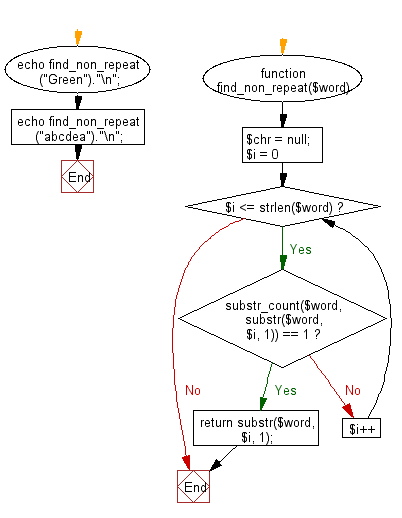﻿ PHP Exercise: Find the first non-repeated character in a given string - w3resource# PHP Exercises: Find the first non-repeated character in a given string

## PHP: Exercise-42 with Solution

Write a PHP program to find the first non-repeated character in a given string.

Sample Example:

Input: Green
Output: G
Input: abcdea
Output: b

Sample Solution: -

PHP Code:

``````<?php
function find_non_repeat(\$word) {
\$chr = null;
for (\$i = 0; \$i <= strlen(\$word); \$i++) {
if (substr_count(\$word, substr(\$word, \$i, 1)) == 1) {
return substr(\$word, \$i, 1);
}
}
}

echo find_non_repeat("Green")."\n";
echo find_non_repeat("abcdea")."\n";
?>
```
```

Sample Output:

```G
b
```

Flowchart:PHP Code Editor:

Have another way to solve this solution? Contribute your code (and comments) through Disqus.

What is the difficulty level of this exercise?

﻿

## PHP: Tips of the Day

How do I strip all spaces out of a string in PHP?

Do you just mean spaces or all whitespace?

For just spaces, use str_replace:

`\$string = str_replace(' ', '', \$string);`

For all whitespace (including tabs and line ends), use preg_replace:

`\$string = preg_replace('/\s+/', '', \$string);`

Ref : https://bit.ly/2Cnb3QZ